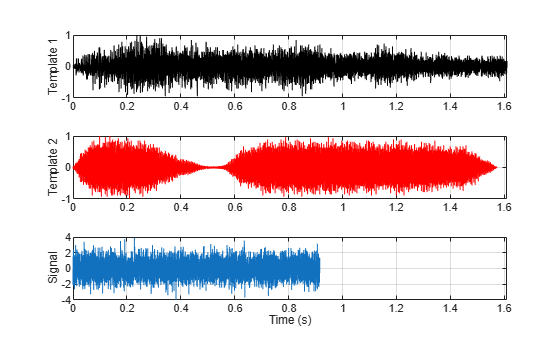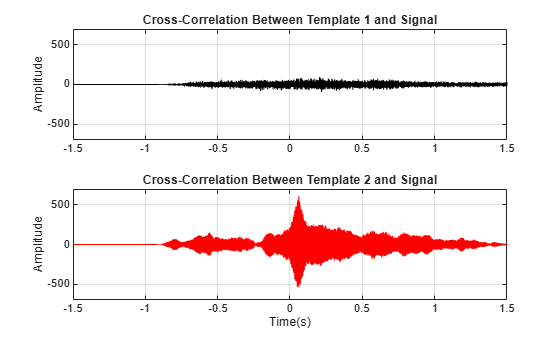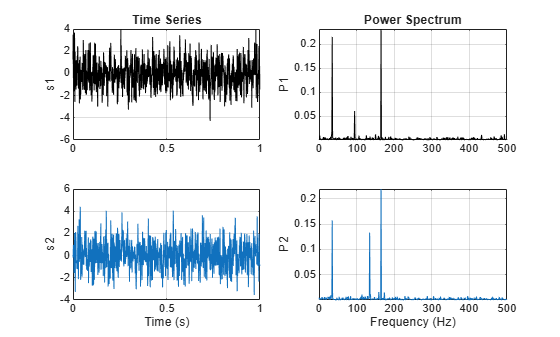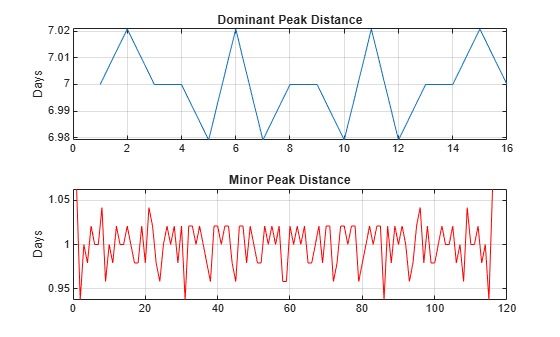# Measure Signal Similarities

This example shows how to measure signal similarities. It will help you answer questions such as: How do I compare signals with different lengths or different sample rates? How do I find if there is a signal or just noise in a measurement? Are two signals related? How do I measure a delay between two signals (and how do I align them)? How do I compare the frequency content of two signals? Similarities can also be found in different sections of a signal to determine if a signal is periodic.

### Compare Signals with Different Sample Rates

Consider a database of audio signals and a pattern matching application where you need to identify a song as it is playing. Data is commonly stored at a low sample rate to occupy less memory.

```load relatedsig figure ax(1) = subplot(3,1,1); plot((0:numel(T1)-1)/Fs1,T1,"k") ylabel("Template 1") grid on ax(2) = subplot(3,1,2); plot((0:numel(T2)-1)/Fs2,T2,"r") ylabel("Template 2") grid on ax(3) = subplot(3,1,3); plot((0:numel(S)-1)/Fs,S) ylabel("Signal") grid on xlabel("Time (s)") linkaxes(ax(1:3),"x") axis([0 1.61 -4 4])```The first and the second subplots show the template signals from the database. The third subplot shows the signal that we want to search for in our database. Just by looking at the time series, the signal does not seem to match to any of the two templates. A closer inspection reveals that the signals actually have different lengths and sample rates.

`[Fs1 Fs2 Fs]`
```ans = 1×3 4096 4096 8192 ```

Different lengths prevent you from calculating the difference between two signals but this can easily be remedied by extracting the common part of signals. Furthermore, it is not always necessary to equalize lengths. Cross-correlation can be performed between signals with different lengths, but it is essential to ensure that they have identical sample rates. The safest way to do this is to resample the signal with a lower sample rate. The `resample` function applies an anti-aliasing (low-pass) FIR filter to the signal during the resampling process.

```[P1,Q1] = rat(Fs/Fs1); % Rational fraction approximation [P2,Q2] = rat(Fs/Fs2); % Rational fraction approximation T1 = resample(T1,P1,Q1); % Change sample rate by rational factor T2 = resample(T2,P2,Q2); % Change sample rate by rational factor```

### Find Signal in Measurement

We can now cross-correlate signal `S` to templates `T1` and `T2` with the `xcorr` function to determine if there is a match.

```[C1,lag1] = xcorr(T1,S); [C2,lag2] = xcorr(T2,S); figure ax(1) = subplot(2,1,1); plot(lag1/Fs,C1,"k") ylabel("Amplitude") grid on title("Cross-Correlation Between Template 1 and Signal") ax(2) = subplot(2,1,2); plot(lag2/Fs,C2,"r") ylabel("Amplitude") grid on title("Cross-Correlation Between Template 2 and Signal") xlabel("Time(s)") axis(ax(1:2),[-1.5 1.5 -700 700])```The first subplot indicates that signal `S` and template `T1` are less correlated, while the high peak in the second subplot indicates that the signal is present in the second template.

```[~,I] = max(abs(C2)); SampleDiff = lag2(I)```
```SampleDiff = 499 ```
`timeDiff = SampleDiff/Fs`
```timeDiff = 0.0609 ```

The peak of the cross-correlation implies that the signal is present in template `T2` starting after 61 ms. In other words, template `T2` leads signal `S` by 499 samples as indicated by `SampleDiff`. This information can be used to align the signals.

### Measure Delay Between Signals and Align Them

Consider a situation where you are collecting data from different sensors recording vibrations caused by cars on both sides of a bridge. When you analyze the signals, you may need to align them. Assume you have 3 sensors working at the same sample rates and measuring signals caused by the same event.

```figure ax(1) = subplot(3,1,1); plot(s1) ylabel("s1") grid on ax(2) = subplot(3,1,2); plot(s2,"k") ylabel("s2") grid on ax(3) = subplot(3,1,3); plot(s3,"r") ylabel("s3") grid on xlabel("Samples") linkaxes(ax,"xy")```We can also use the `finddelay` function to find the delay between two signals.

`t21 = finddelay(s1,s2)`
```t21 = -350 ```
`t31 = finddelay(s1,s3)`
```t31 = 150 ```

`t21` indicates that `s2` lags `s1` by 350 samples, and `t31` indicates that `s3` leads `s1` by 150 samples. This information can now be used to align the 3 signals by time shifting the signals. We can also use the `alignsignals` function to align the signals by delaying the earliest signal.

```s1 = alignsignals(s1,s3); s2 = alignsignals(s2,s3); figure ax(1) = subplot(3,1,1); plot(s1) grid on title("s1") axis tight ax(2) = subplot(3,1,2); plot(s2) grid on title("s2") axis tight ax(3) = subplot(3,1,3); plot(s3) grid on title("s3") axis tight linkaxes(ax,"xy")```### Compare Frequency Content of Signals

A power spectrum displays the power present in each frequency. Spectral coherence identifies frequency-domain correlation between signals. Coherence values tending towards 0 indicate that the corresponding frequency components are uncorrelated while values tending towards 1 indicate that the corresponding frequency components are correlated. Consider two signals and their respective power spectra.

```Fs = FsSig; % Sample Rate [P1,f1] = periodogram(sig1,[],[],Fs,"power"); [P2,f2] = periodogram(sig2,[],[],Fs,"power"); figure t = (0:numel(sig1)-1)/Fs; subplot(2,2,1) plot(t,sig1,"k") ylabel("s1") grid on title("Time Series") subplot(2,2,3) plot(t,sig2) ylabel("s2") grid on xlabel("Time (s)") subplot(2,2,2) plot(f1,P1,"k") ylabel("P1") grid on axis tight title("Power Spectrum") subplot(2,2,4) plot(f2,P2) ylabel("P2") grid on axis tight xlabel("Frequency (Hz)")```The `mscohere` function calculates the spectral coherence between the two signals. It confirms that `sig1` and `sig2` have two correlated components around 35 Hz and 165 Hz. In frequencies where spectral coherence is high, the relative phase between the correlated components can be estimated with the cross-spectrum phase.

```[Cxy,f] = mscohere(sig1,sig2,[],[],[],Fs); Pxy = cpsd(sig1,sig2,[],[],[],Fs); phase = -angle(Pxy)/pi*180; [pks,locs] = findpeaks(Cxy,MinPeakHeight=0.75); figure subplot(2,1,1) plot(f,Cxy) title("Coherence Estimate") grid on hgca = gca; hgca.XTick = f(locs); hgca.YTick = 0.75; axis([0 200 0 1]) subplot(2,1,2) plot(f,phase) title("Cross-Spectrum Phase (deg)") grid on hgca = gca; hgca.XTick = f(locs); hgca.YTick = round(phase(locs)); xlabel("Frequency (Hz)") axis([0 200 -180 180])```The phase lag between the 35 Hz components is close to -90 degrees, and the phase lag between the 165 Hz components is close to -60 degrees.

### Find Periodicities in Signal

Consider a set of temperature measurements in an office building during the winter season. Measurements were taken every 30 minutes for about 16.5 weeks.

```load officetemp.mat Fs = 1/(60*30); % Sample rate is 1 sample every 30 minutes days = (0:length(temp)-1)/(Fs*60*60*24); figure plot(days,temp) title("Temperature Data") xlabel("Time (days)") ylabel("Temperature (Fahrenheit)") grid on```With the temperatures in the low 70s, you need to remove the mean to analyze small fluctuations in the signal. The `xcov` function removes the mean of the signal before computing the cross-correlation and returns the cross-covariance. Limit the maximum lag to 50% of the signal to get a good estimate of the cross-covariance.

```maxlags = numel(temp)*0.5; [xc,lag] = xcov(temp,maxlags); [~,df] = findpeaks(xc,MinPeakDistance=5*2*24); [~,mf] = findpeaks(xc); figure plot(lag/(2*24),xc,"k",... lag(df)/(2*24),xc(df),"kv",MarkerFaceColor="r") grid on xlim([-15 15]) xlabel("Time (days)") title("Auto-Covariance")```Observe dominant and minor fluctuations in the auto-covariance. Dominant and minor peaks appear equidistant. To verify if they are, compute and plot the difference between the locations of subsequent peaks.

```cycle1 = diff(df)/(2*24); cycle2 = diff(mf)/(2*24); subplot(2,1,1) plot(cycle1) ylabel("Days") grid on title("Dominant Peak Distance") subplot(2,1,2) plot(cycle2,"r") ylabel("Days") grid on title("Minor Peak Distance")````mean(cycle1)`
```ans = 7 ```
`mean(cycle2)`
```ans = 1 ```

The minor peaks indicate 7 cycles/week and the dominant peaks indicate 1 cycle/week. This makes sense given that the data comes from a temperature-controlled building on a 7-day calendar. The first 7-day cycle indicates that there is weekly cyclic behavior of the building temperature where temperatures lower during the weekends and go back to normal during the week days. The 1-day cycle behavior indicates that there is also daily cyclic behavior where temperatures lower during the night and increase during the day.